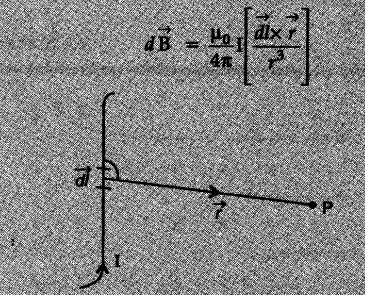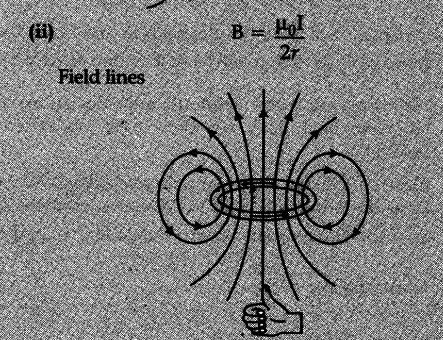# (i) State Biot-Savart law in vector form expressing the magnetic field due to an element dl carrying current I at a distance r from the element

(i) State Biot-Savart law in vector form expressing the magnetic field due to an element dl carrying current I at a distance r from the element
(ii) Write the expression for the magnitude of the magnetic field at the centre of a Circular loop of radius r carrying a steady current I. Draw the field lines due to the current loop.

According to Biot-Savart’s jaw, the magnetic field due to a current element dl carrying current
l at a point P with position vector r is given by